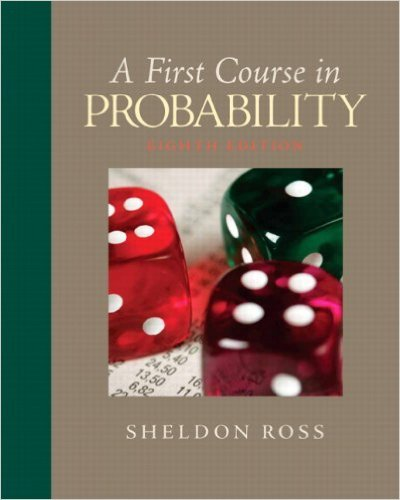×

×

# Solutions for Chapter 4: Random Variables## Full solutions for First Course in Probability | 8th Edition

ISBN: 9780136033134Solutions for Chapter 4: Random Variables

Solutions for Chapter 4
4 5 0 264 Reviews
17
3
##### ISBN: 9780136033134

Summary of Chapter 4: Random Variables

First Course in Probability was written by and is associated to the ISBN: 9780136033134. Chapter 4: Random Variables includes 85 full step-by-step solutions. Since 85 problems in chapter 4: Random Variables have been answered, more than 26609 students have viewed full step-by-step solutions from this chapter. This expansive textbook survival guide covers the following chapters and their solutions. This textbook survival guide was created for the textbook: First Course in Probability, edition: 8.

Key Statistics Terms and definitions covered in this textbook
• 2 k p - factorial experiment

A fractional factorial experiment with k factors tested in a 2 ? p fraction with all factors tested at only two levels (settings) each

• a-error (or a-risk)

In hypothesis testing, an error incurred by failing to reject a null hypothesis when it is actually false (also called a type II error).

• Additivity property of x 2

If two independent random variables X1 and X2 are distributed as chi-square with v1 and v2 degrees of freedom, respectively, Y = + X X 1 2 is a chi-square random variable with u = + v v 1 2 degrees of freedom. This generalizes to any number of independent chi-square random variables.

• All possible (subsets) regressions

A method of variable selection in regression that examines all possible subsets of the candidate regressor variables. Eficient computer algorithms have been developed for implementing all possible regressions

• Analytic study

A study in which a sample from a population is used to make inference to a future population. Stability needs to be assumed. See Enumerative study

• Bayes’ theorem

An equation for a conditional probability such as PA B ( | ) in terms of the reverse conditional probability PB A ( | ).

• Bimodal distribution.

A distribution with two modes

• Causal variable

When y fx = ( ) and y is considered to be caused by x, x is sometimes called a causal variable

• Center line

A horizontal line on a control chart at the value that estimates the mean of the statistic plotted on the chart. See Control chart.

• Combination.

A subset selected without replacement from a set used to determine the number of outcomes in events and sample spaces.

• Completely randomized design (or experiment)

A type of experimental design in which the treatments or design factors are assigned to the experimental units in a random manner. In designed experiments, a completely randomized design results from running all of the treatment combinations in random order.

• Components of variance

The individual components of the total variance that are attributable to speciic sources. This usually refers to the individual variance components arising from a random or mixed model analysis of variance.

• Consistent estimator

An estimator that converges in probability to the true value of the estimated parameter as the sample size increases.

• Contingency table.

A tabular arrangement expressing the assignment of members of a data set according to two or more categories or classiication criteria

• Continuous distribution

A probability distribution for a continuous random variable.

• Cumulative normal distribution function

The cumulative distribution of the standard normal distribution, often denoted as ?( ) x and tabulated in Appendix Table II.

• Enumerative study

A study in which a sample from a population is used to make inference to the population. See Analytic study

• Error sum of squares

In analysis of variance, this is the portion of total variability that is due to the random component in the data. It is usually based on replication of observations at certain treatment combinations in the experiment. It is sometimes called the residual sum of squares, although this is really a better term to use only when the sum of squares is based on the remnants of a model-itting process and not on replication.

• Exponential random variable

A series of tests in which changes are made to the system under study

• Extra sum of squares method

A method used in regression analysis to conduct a hypothesis test for the additional contribution of one or more variables to a model.